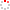IMR OpenIR
 Mathematical structure of the three-dimensional (3D) Ising model Z. D. Zhang 2013 Source Publication Chinese Physics BISSN 1674-1056 Volume 22Issue:3 Abstract An overview of the mathematical structure of the three-dimensional (3D) Ising model is given from the points of view of topology, algebra, and geometry. By analyzing the relationships among transfer matrices of the 3D Ising model, Reidemeister moves in the knot theory, Yang-Baxter and tetrahedron equations, the following facts are illustrated for the 3D Ising model. 1) The complex quaternion basis constructed for the 3D Ising model naturally represents the rotation in a (3+1)-dimensional space-time as a relativistic quantum statistical mechanics model, which is consistent with the 4-fold integrand of the partition function obtained by taking the time average. 2) A unitary transformation with a matrix that is a spin representation in 2(n.l.o)-space corresponds to a rotation in 2n . l . o-space, which serves to smooth all the crossings in the transfer matrices and contributes the non-trivial topological part of the partition function of the 3D Ising model. 3) A tetrahedron relationship would ensure the commutativity of the transfer matrices and the integrability of the 3D Ising model, and its existence is guaranteed by the Jordan algebra and the Jordan-von Neumann-Wigner procedures. 4) The unitary transformation for smoothing the crossings in the transfer matrices changes the wave functions by complex phases phi(x), phi(y), and phi(z). The relationship with quantum field and gauge theories and the physical significance of the weight factors are discussed in detail. The conjectured exact solution is compared with numerical results, and the singularities at/near infinite temperature are inspected. The analyticity in beta = 1/(k(B)T) of both the hard-core and the Ising models has been proved only for beta > 0, not for beta = 0. Thus the high-temperature series cannot serve as a standard for judging a putative exact solution of the 3D Ising model. description.department chinese acad sci, inst met res, shenyang natl lab mat sci, shenyang 110016, peoples r china. ; zhang, zd (reprint author), chinese acad sci, inst met res, shenyang natl lab mat sci, shenyang 110016, peoples r china. ; zdzhang@imr.ac.cn Keyword Ising Model Topology Algebra Geometry Recent Conjectured Solution Star-triangle Relation Vapor Critical-point Quantum-field Theory Critical Exponents Tetrahedron Equation 2-dimensional Model Integrable Models Lattice Models Ground-state URL 查看原文 Language 英语 Document Type 期刊论文 Identifier http://ir.imr.ac.cn/handle/321006/71701 Collection 中国科学院金属研究所 Recommended CitationGB/T 7714 Z. D. Zhang. Mathematical structure of the three-dimensional (3D) Ising model[J]. Chinese Physics B,2013,22(3). APA Z. D. Zhang.(2013).Mathematical structure of the three-dimensional (3D) Ising model.Chinese Physics B,22(3). MLA Z. D. Zhang."Mathematical structure of the three-dimensional (3D) Ising model".Chinese Physics B 22.3(2013).
 Files in This Item: There are no files associated with this item.
 Related Services Recommend this item Bookmark Usage statistics Export to Endnote Google Scholar Similar articles in Google Scholar [Z. D. Zhang]'s Articles Baidu academic Similar articles in Baidu academic [Z. D. Zhang]'s Articles Bing Scholar Similar articles in Bing Scholar [Z. D. Zhang]'s Articles Terms of Use No data! Social Bookmark/Share